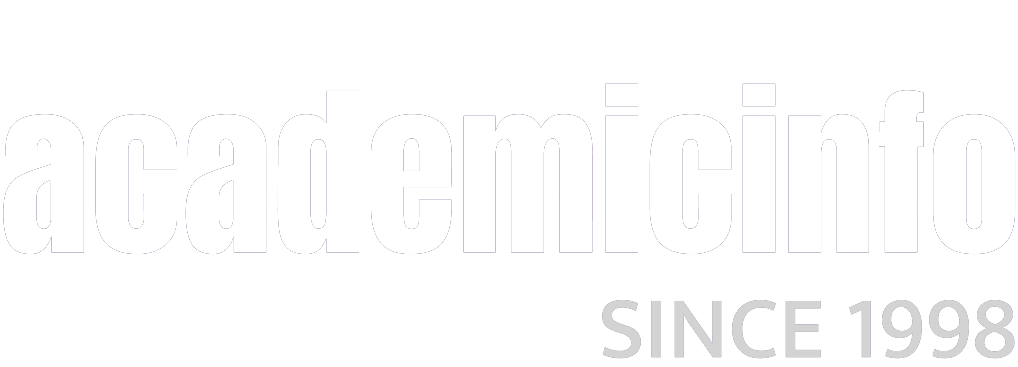# Calculas Resources

Sciences > Mathematics> Calculus

BrainTrax
"Our materials are written for the struggling student. We put the concepts in language they can understand. We also use this navigation tool (the Brain) because it associates math concepts for the student. For whatever reason, it appears that our system works, and makes a difference in the student success rate (in lower level math courses, anyway) at our university... Additionally, we have determined that students who use our system in junior-high and senior-high level algebra instruction can also benefit significantly from using the BrainTrax System."
Sections include: Algebra ; Trigonometry ; Calculus I ; Calculus II.
- University of Missouri, Rolla

Calculus@internet
A very extensive annotated listing of calculus resources.
Calculus nets include labs, differential, homework, integral,
VRML, vector, linear, ode, texts, projects.

Calculus-Help.com
Formerly the Kelly AP Calculus Homepage
By Mike Kelly, author of Master the AP Calculus AB
and BC Tests

calculus.org: THE CALCULUS PAGE
Sections include: Resources For the Calculus Student ; Resources For the Calculus Instructor ; Links ; Prizes and Competitions.
Edited by Joel Hass and Duane Kouba, Dept. of Mathematics, University of California, Davis.

Common Errors in College Math
By Eric Schechter, Mathematics Dept., Vanderbilt University

Graphics for the calculus classroom
Douglas N. Arnold, Professor of Mathematics, Penn State University

HMC Calculus Tutorial
Sections include: Pre-Calculus ; Single Variable Calculus ; Multi-Variable Calculus ; Linear Algebra ; Differential Equations.
- Harvey Mudd College - Department of Mathematics

Karl's Calculus Tutor
"Enter the tutorial (below) or search this website for a calculus topic. You will find coverage of limits, continuity, derivatives, related rates, optimization, L'Hopital's rule, integration, and much more. There are dozens of problems worked out for you step-by-step."
By Karl Hahn

Math Archives - Calculus Resources On-Line
Sections include: Initiatives & Programs ; Articles & Discussions ; Software ; Publisher Sites ; Other Listings.
By Przemyslaw Bogacki

Math Forum: Calculus
Sections include: Classroom Materials for Teachers and Students ; Software ; Internet Projects ; Public Forums.
From The Math Fourm @ Drexel

The MathServ Calculus Toolkit
Sections include: Factoring Polynomials ; Partial Fractions ; Polynomial Equations ; Graph of Functions ; Graphs of Equations ; Limits ; Derivatives ; Antiderivaties ; Definite Integrals ; Inverse Functions ; Newton's Method ; Polynomial Interpolation ; Sums ; Parametric Equations ; Polar Functions.
By Crooke & Tschantz, Vanderbilt University

MathWorld - Eric Weisstein's World of Mathematics
Subjects include: Algebra ; Applied Mathematics ; Calculus and Analysis ; Discrete Mathematics ; Foundations of Mathematics ; Geometry ; History and Terminology ; Number Theory ; Probability and Statistics ; Recreational Mathematics ; Topology.

NetMath
"NetMath allows high school students, home-schooled students, and adult/college-level students (including the deaf) to learn calculus, earn college credit, and gain other skills to advance their education..."
University of Illinois

Numerical Methods for Partial Differential Equations: An Overview and Applications
"This is the web edition of the 3-4 weeks course F2A5076 taught 1997-2001 at the Royal Institute of Technology in Stockholm (Sweden). The main target is to provide a robust introduction in computational methods to graduate- and lifelong learning students, using a distance learning method that can easily be tailored to professional schedules."

SimCalc Project: Simulations for Calculus Learning
"Aims to democratize access to the Mathematics of Change for mainstream students by combining advanced simulation technology with innovative curriculum that begins in the early grades and includes powerful ideas extending beyond classical calculus."
University of Massachusetts, Dartmouth; and TERC, Cambridge, MA.

Tutorial on Optimization Theory and Difference and Differential Equations
"This tutorial is a hypertext version of my lecture notes for a course in optimization theory and difference and differential equations...The tutorial is intended to be a complement for a book rather than a substitute."
By Martin J. Osborne, Dept. of Economics, University of Toronto.

Visual Calculus
"A collection of modules that can be used in the studying or teaching calculus."
The tutorials include Pre-Calculus
By Lawrence S. Husch, Mathematics Dept, University of Tennessee, Knoxville